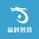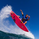# Spiky Iguana Multi RSI Bands (Reverse RSI for the Price) by RRB

317 views
317
Spiky Iguana Multi RSI Bands by RagingRocketBull 2018
Version 1.0

This indicator shows multiple RSI Bands with prices corresponding to specified overbought/oversold RSI levels.
It is used to extrapolate the exact price levels currently matching a given set of RSI levels based on prior price/RSI levels action.
You can think of it as a reverse RSI where RSI levels are moving dynamically around the price instead of price bouncing between straight lines.

Features:
- 6+6 = 12 customizable Overbought(R)/Oversold(S) RSI Levels + 6 Mean(M) lines
- Multicolor levels/fill ranges
- Show/Hide specific S/R/M levels and fill ranges

1. uses plot*, fill and is based on RSIBANDS_LB

Good Luck! Feel free to explore and learn from the codeVery beautiful code.
x = (len - 1) * ( adc * level / (100-level) - auc)
I don't quite understand this sentence. What logic is it based on? Can you explain it? Thx.
Antwortenthis indicator is based on RSIBANDS_LB. Perhaps, LB himself can better explain how reverse RSI is calculated. I modified his code to add more levels.

RSI Levels are specified in percentages , so some sort of normalization is needed for x - that's what that line does.
auc = AvgU = average of all up moves in the last ep price bars
adc = AvgD = average of all down moves in the last ep price bars

This is how RSI is calculated (script uses the same formula):